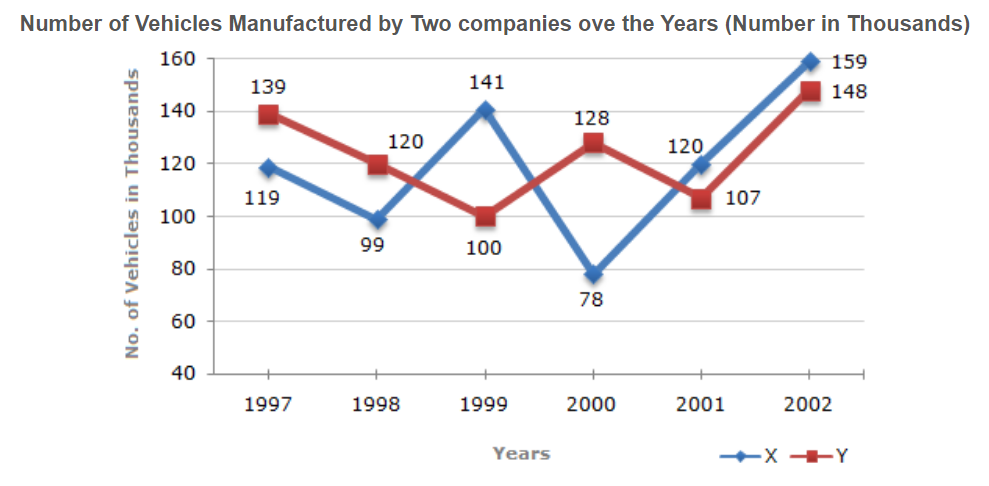# E-PolyLearning

 11. Study the following line graph and answer the question based on it. What is the difference between the number of vehicles manufactured by Company Y in 2000 and 2001 ?a. 50000 b. 42000 c. 33000 d. 21000

 12. Study the following line graph and answer the question based on it. What is the difference between the total productions of the two Companies in the given years ?a. 19000 b. 22000 c. 26000 d. 28000
 13. Study the following line graph and answer the question based on it. What is the average numbers of vehicles manufactured by Company X over the given period ? (rounded off to nearest integer)a. 119333 b. 113666 c. 112778 d. 111223
 14. Study the following line graph and answer the question based on it. In which of the following years, the difference between the productions of Companies X and Y was the maximum among the given years ?a. 1997 b. 1998 c. 1999 d. 2000
 15. Study the following line graph and answer the question based on it. The production of Company Y in 2000 was approximately what percent of the production of Company X in the same year ?a. 173 b. 164 c. 132 d. 97